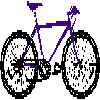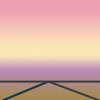#### You may also likeFour vehicles travelled on a road. What can you deduce from the times that they met?### Enclosing Squares

Can you find sets of sloping lines that enclose a square?### Intersections

Change one equation in this pair of simultaneous equations very slightly and there is a big change in the solution. Why?

# Painting Between the Lines

### Why do this problem?

This problem gives an engaging opportunity for genuine cross-curricular links between art and mathematics whilst bringing in ideas about the equations of straight line graphs. By considering pictures made up of curves as well as lines there are natural extensions into transformation of simple functions, and thinking about how to describe shaded parts can lead into work on inequalities.

### Possible approach

In working on this problem learners will need to be fluent in expressing equations of straight lines - Diamond Collector offers a good exercise to practise this.

Learners could be set a homework task before the lesson of finding an image which could be represented in an abstract way using straight lines (or very simple curves such as parabolas) - the photo and the abstract image used in the problem could be given as an example.

Begin by discussing with the class the abstract image of the pyramids. The image could be projected onto a whiteboard and the class could decide together on an origin and scale for the axes. Ask them to discuss in pairs how they would write a set of mathematical instructions to create the image (or how they would create it using graphing software), and then share ideas with the whole class.

Key concepts to draw out would be the use of equations of straight lines, using inequalities to express which parts of a line to draw as line segments, using inequalities to describe which sections to colour in each shade, and where the class would choose to put the axes.

Now hand out this worksheet (Word, PDF) which contains instructions for drawing another well-known landmark. Suggest that learners work in pairs to complete the image. One possible extension at this point is for them to devise a way of using inequalities to describe a way of colouring the picture.

Then give the class plenty of time to take an image of their own and turn it into an abstract image on squared paper with axes, perhaps working in pairs again. Learners who have met equations of quadratic graphs could create an image using parabolas as well as straight lines. They could then write out a set of instructions to draw their image and challenge another pair to recreate it without seeing the original. Graphing software could be used to verify the equations of lines that learners choose to use.

Another approach could be to give everyone the same image to recreate using lines and simple curves, and then liaise with some teachers and students of art to choose which is the best abstract representation of the original image.

### Key questions

How can the image be recreated using only straight lines?